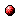# Approximate dynamic programming with a fuzzy parameterization

Reference:
L. Busoniu, D. Ernst, B. De Schutter, and R. Babuska, "Approximate dynamic programming with a fuzzy parameterization," Automatica, vol. 46, no. 5, pp. 804-814, May 2010.

Abstract:
Dynamic programming (DP) is a powerful paradigm for general, nonlinear optimal control. Computing exact DP solutions is in general only possible when the process states and the control actions take values in a small discrete set. In practice, it is necessary to approximate the solutions. Therefore, we propose an algorithm for approximate DP that relies on a fuzzy partition of the state space, and on a discretization of the action space. This fuzzy Q-iteration algorithm works for deterministic processes, under the discounted return criterion. We prove that fuzzy Q-iteration asymptotically converges to a solution that lies within a bound of the optimal solution. A bound on the suboptimality of the solution obtained in a finite number of iterations is also derived. Under continuity assumptions on the dynamics and on the reward function, we show that fuzzy Q-iteration is consistent, i.e., that it asymptotically obtains the optimal solution as the approximation accuracy increases. These properties hold both when the parameters of the approximator are updated in a synchronous fashion, and when they are updated asynchronously. The asynchronous algorithm is proven to converge at least as fast as the synchronous one. The performance of fuzzy Q-iteration is illustrated in a two-link manipulator control problem.

Downloads:Online version of the paperCorresponding technical report: pdf file (1.49 MB)
Note: More information on the pdf file format mentioned above can be found here.

Bibtex entry:

@article{BusErn:09-047,
author={L. Bu{\c{s}}oniu and D. Ernst and B. {D}e Schutter and R. Babu{\v{s}}ka},
title={Approximate dynamic programming with a fuzzy parameterization},
journal={Automatica},
volume={46},
number={5},
pages={804--814},
month=may,
year={2010},
doi={10.1016/j.automatica.2010.02.006}
}

Go to the publications overview page.

This page is maintained by Bart De Schutter. Last update: December 29, 2019.